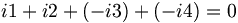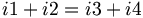# Kirchhoff's Current and Voltage Laws

(diff) ← Older revision | Latest revision (diff) | Newer revision → (diff)

## Kirchhoff's Current Law

Kirchhoff's Current Law says that the current going into a junction is equal to the current going out of a junction. In other words, the sum of the currents entering the node must be zero (if we consider currents leaving the node to be a negative current entering the node). Consider the following diagram:

For the node A in the center, i1 and i2 are entering the node, and i3 and i4 are leaving the node. We would write:$i1+i2+(-i3)+(-i4)=0\,$ which can also be written as$i1+i2=i3+i4\,$

Note that i7 is equal to i2; we can prove this by analyzing node B. We can also treat everything between node C and D as one big node, and conclude that i5 is equal to i6 without having to know the value of any of the currents within.